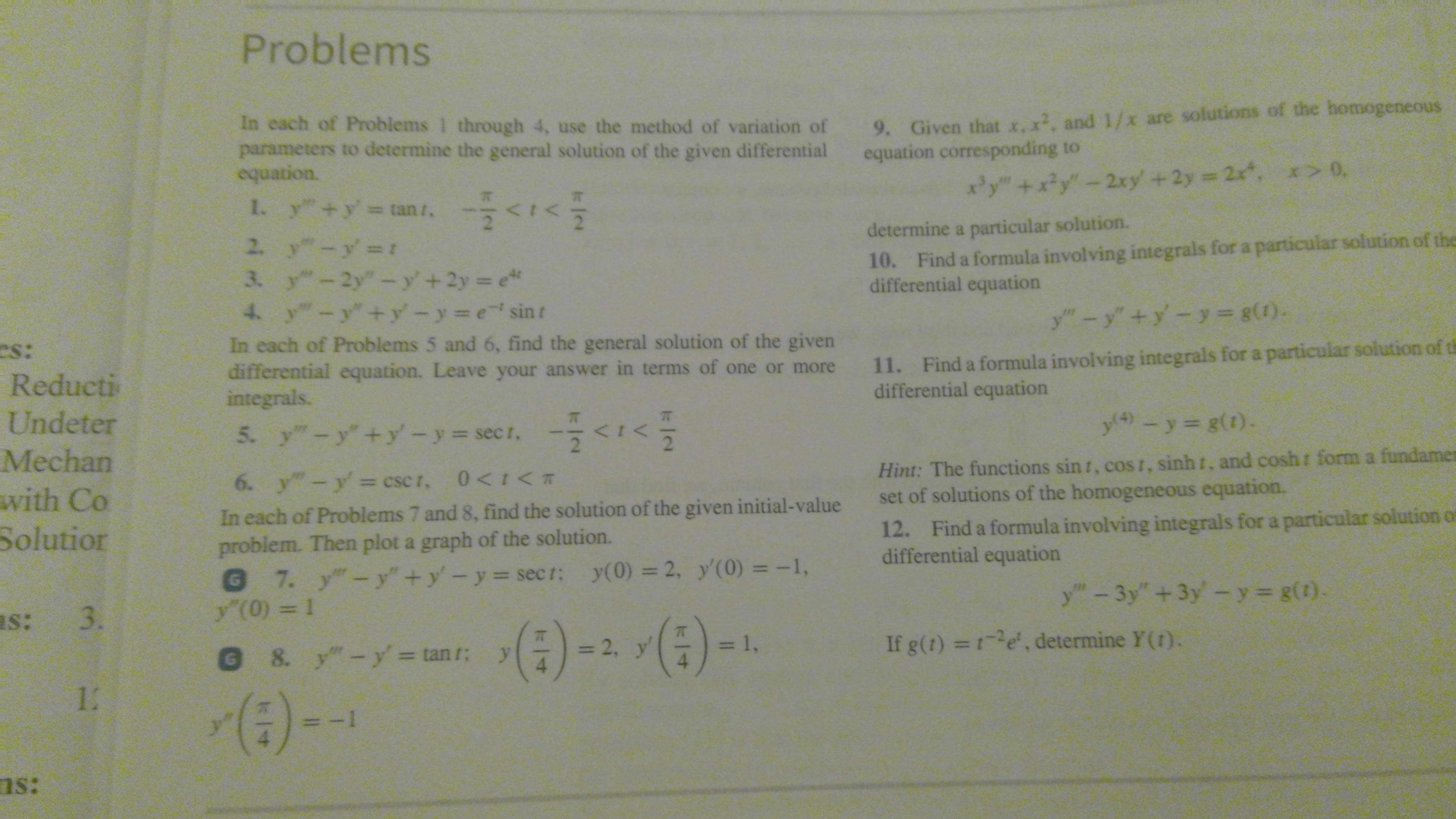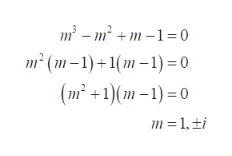# Problems9. Given that x, x2, and 1/x are solutions of the homogeneousequation corresponding toIn cach of Problems 1 through 4, use the method of variation ofparameters to determine the general solution of the given differentialequation.xy"+x?y"-2xy +2y 2x, x> 0,1. y +y tan t,determine a particular solution.10. Find a formula involving integrals for a particular solution of thedifferential equation2. y"-y' t3. y-2y"-y'+2y e4. y-y"+y'-y e sinty"-y"+y-y= g(1).In each of Problems 5 and 6, find the general solution of the givendifferential equation. Leave your answer in terms of one or moreintegrals.11. Find a formula involving integrals for a particular solution of thdifferential equationes:ReductiUndetery4)- y= g(1).5. y-y+y-y-secr, -

Question
4 views

How might I be able to answer problem 4? This problem is from a Differential Equations Textbook. The section is titled, "The Method of Variation of Parameters."help_outlineImage TranscriptioncloseProblems 9. Given that x, x2, and 1/x are solutions of the homogeneous equation corresponding to In cach of Problems 1 through 4, use the method of variation of parameters to determine the general solution of the given differential equation. xy"+x?y"-2xy +2y 2x, x> 0, 1. y +y tan t, determine a particular solution. 10. Find a formula involving integrals for a particular solution of the differential equation 2. y"-y' t 3. y-2y"-y'+2y e 4. y-y"+y'-y e sint y"-y"+y-y= g(1). In each of Problems 5 and 6, find the general solution of the given differential equation. Leave your answer in terms of one or more integrals. 11. Find a formula involving integrals for a particular solution of th differential equation es: Reducti Undeter y4) - y= g(1). 5. y-y+y-y-secr, -<1< 2 Hint: The functions sin t, cost, sinh t, and cosh set of solutions of the homogeneous equation. Mechan form a fundamen 6. y"-y= csc t, 0
check_circle

Step 1

In the method of variation of method, the complete solution is calculated just by the CF, NO PI is needed.

Step 2

For the differential equation, y’’’y’’+y’-y=e-tsint the characteristic equation is,help_outlineImage Transcriptionclosem – m² + m –1= 0 m² (m–1)+1(m –1) = 0 (m² +1)(m – 1) = 0 m = 1, ±i fullscreen
Step 3

For m=1,i, -i the yc(t)...

### Want to see the full answer?

See Solution

#### Want to see this answer and more?

Solutions are written by subject experts who are available 24/7. Questions are typically answered within 1 hour.*

See Solution
*Response times may vary by subject and question.
Tagged in

### Math